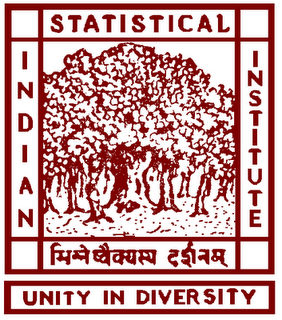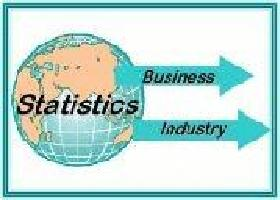# Statistical Methods in Finance 2017

Dec 16 - 19, 2017## Abstract

Modelling Time Series Through Gini Autocovariance Function

by Sudheesh K Kattumannil

Measuring statistical dependence of an observed time series is an important problem in many areas diverse from statistical signal processing to financial modeling. In stationary time series modelling, autocovariance function (ACV) and associated autocorrelation function are extensively used to describe the dependence structure but the existence of the second moment is preassumed. Moreover, these measures quantify only partial dependence when the time series is nonlinear or innovations are non-Gaussian. In this talk, we introduce an alternate way of modelling stationary time series through Gini ACV, which require only the existence of first moment. After introducing the basic concepts, we discuss the recent developments in this area. At the end of the talk we will explore some open problems.